# New Analytical and Numerical Solutions of the Particle Breakup Process

Abdelmalek Hasseine1, *, Mark W. Hlawitschka2, Waid Omar3, Hans-Jörg Bart2
1 Laboratory of Civil Engineering, Hydraulic, Sustainable Development and Environmental, University of Biskra, Biskra, Algeria
2 Chair of Separation Science and Technology, TU Kaiserslautern, P.O. Box 3049, D-67653 Kaiserslautern, Germany
3 Al-Balqa Applied University, Faculty of Engineering Technology, Department of Chemical Engineering P.O. Box 15008, Marka 11134, Jordan

© 2020 Hasseine et al.

open-access license: This is an open access article distributed under the terms of the Creative Commons Attribution 4.0 International Public License (CC-BY 4.0), a copy of which is available at: https://creativecommons.org/licenses/by/4.0/legalcode. This license permits unrestricted use, distribution, and reproduction in any medium, provided the original author and source are credited.

* Address correspondence to this author at the Laboratory of Civil Engineering, Hydraulic, Sustainable Development and Environmental, University of Biskra, Biskra, Algeria; E-mail: hasseine@yahoo.fr

## Abstract

### Objective:

In this work, we obtained the analytical and approximate solutions of the population balance equations (PBEs) involving the breakup process in batch and continuous flow by applying the Adomian decomposition method and piecewise continuous basis functions, respectively.

### Methods:

The key to the advanced numerical method is to represent the number distribution function of the dispersed phase through the orthogonal Chebyshev basis polynomials. It is a straightforward and effective method that has the advantage of simultaneously giving the distribution and the different required moments. Therefore, it does not require the construction of the distribution from moments computations obtained by the transformation of the initial problem and the lost information.

### Results:

The performance of this numerical approach is evaluated by solving breakup equation and comparison against analytical solutions obtained from the Adomian decomposition method, which generally allows the analysis of this approach.

### Conclusion:

The numerical results obtained by the present numerical method were compared with the new analytical solutions of the PBE. It was found that both piecewise continuous basis functions and analytical solutions have comparable results.

Keywords: Population balance, Decomposition method, Piecewise continuous solution, Bioreactors, Monte Carlo methods, Nanoparticle physics.

## 1. INTRODUCTION

Population balance models of dispersed phases find many scientific and engineering applications including liquid-liquid, liquid-vapor and solid-liquid dispersions, nanoparticle physics, pharmaceutical industries, polymerization and bioreactors [1-11].

The complex structure of the PBE allows for an analytical solution only for some simple breakup kernels [8-10]. Several approximate resolution methods, such as weighted residuals [1-7], successive approximations , finite elements techniques [9, 13-16], finite volume scheme [17-22], fixed and moving pivot approaches , Monte Carlo methods [24-26] and method of moments with these several extensions such as QMOM, DQMOM, SQMOM and EQMOM [6, 27-29] have been widely studied.

For these moment methods, only a few moments are obtained. Therefore, after having computed these moments, it is necessary to reconstruct the distribution corresponding to the resulting droplet size distributions. Since the droplet size distributions generally constitute the key result to clearly evaluate the quality of the process, and the importance of the reconstruction procedure.

The key disadvantage of the MOM and its basic variants [5, 6, 30] is its incapacity to reconstruct the density function. Contrary to these numerical approaches, piecewise continuous solutions approach proposed in this work effectively and simultaneously provides the distribution and the different required moments without having to express the PBE in using moments.

Consequently, the different moments can be obtained by a simple integration of the solution. It should be noted that the present numerical method shows significant enhancement that avoids expressing population balance equation in terms of moments.

The aim of the present work is to show the advantage of this new proposed numerical method compared to the new analytical solutions of the (PBEs) using the Adomian decomposition method by considering the particle breakup process in batch and continuous flow. The term ‘particles’ might be referred to droplets, bubbles, crystals or grains, and may have several properties like size, composition, porosity, and enthalpy. The population balance equation model and the finite element method with the expansion coefficients approach for solving the breakup process will be described. Finally, the proposed numerical approach is compared with the analytical results for different assumed breakup kernels and physically relevant problems.

This paper is arranged as follows. In section two, we briefly introduce the population balance equation model. Then, in section 3, the finite element method with the expansion coefficients approach for solving the breakup process is described. Finally, the numerical approach is tested using analytical results obtained in this work.

## 1.1. Population Balance Equation for the Breakup Process in Continuous Flow:

The PBE that describes the evolution of the droplet number density,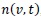in a continuous well-stirred vessel, with droplet formation and loss terms due to breakup, can be expressed as .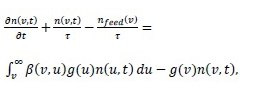(1)

where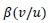is the diameter density distribution of daughter droplets and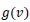is the droplet breakup frequency. The overall input flow determines the residence time τ of the dispersion. Thus, assuming a perfect mixer, where the outlet droplet volume distribution is always identical to the distribution inside of the vessel,, the resulting droplet entry and exit frequencies become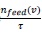and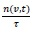, respectively. The two parts in the source term represent the droplet birth due to the generation of new droplets and the second term describes droplet death due to the breakup process.

In this work, the required breakup kernels to close Eq. (1) are shown in Table 1.

## 1.2. The Piecewise Continuous Solution Scheme

We consider the solution of the PBE for the droplet breakup in batch and continuous flow. As discussed in the introduction part, one of the most applied approaches for solving the PBE is the moment method. Alternatively, we present in this section, piecewise continuous solution scheme.

The principal idea of this method is to represent the distribution function as a Chebyshev truncated series as.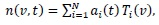(2)

where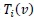, are the Chebyshev polynomials of the first kind of degree i which are orthogonal with respect to the weight function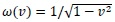on the interval [-1, 1] and satisfy the following recursive formula: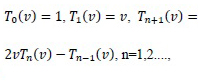(3)

and the unknown Chebyshev coefficients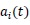remain to be computed.

Insertinginto the PBE (1) and multiplying both sides of the breakup equation by a test function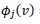and integrating from v=0 to ∞lead to

Table 1. Summary of the test cases used in the PBE to validate the finite element expansion scheme.
Case Process g(d) β(d/u) Feed Distribution Initial Condition
1 Batch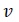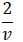0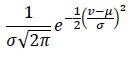2 Batch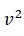03 Continuous0
3 Continuous0
3 Continuous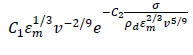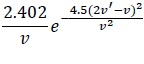0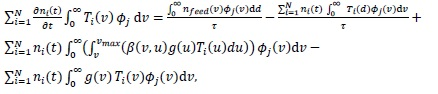(4)

Eq. (4) is then approached by: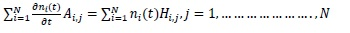(5)

with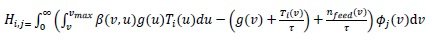(6)

and the mass matrix integral coefficients Ai,j can be written as.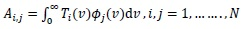(7)

It should be noted in the case of the pure Galerkin approximation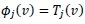and for the collocation approach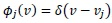, where the shifted Dirac delta functions are with Vj collocation points.

In the case in which the spatial domain is chosen as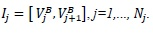. The points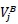and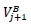are the boundary points for each interval. Let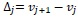be the width of an element and define the center of the interval Ij by Vj and the approximate solution is piecewise a polynomial of degree k then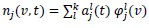, where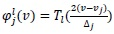and Tl is the lth Chebyshev orthogonal polynomial.

The initial values of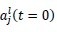are obtained up to order 3 as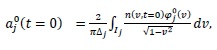(8)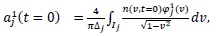(9)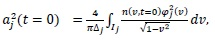(10)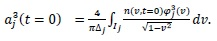(11)

For each interval Ij and each l = 0,..., k, the expansion coefficients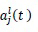are the degrees of freedom.

When the solution is obtained for the expansion coefficients, the kth moment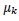of the number density function is then computed easily as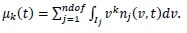(12)

## 2. THE DECOMPOSITION METHOD

To illustrate the basic concepts of the Adomian decomposition method, we consider the following nonlinear differential equation: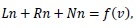(13)

where L is the linear operator chosen as the highest-order derivative, which must be easily invertible. The remainder of the linear operator is R and the nonlinear term is represented by Nn, and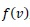is an inhomogeneous term.

Since L is invertible and using the initial condition, we have the canonical form: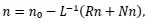(14)

by considering only the linear term, the Adomian decomposition method [31, 32] for the unknown function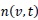can be written as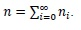(15)

The solution of the linear partial differential equation in the form (14) with the initial value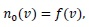(16)

can be obtained by the series (15) with the following recursion scheme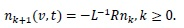(17)

## 2.1. Illustrative Tests Cases

The numerical solution using the finite element expansion coefficients of the breakup equation outlined above in batch and continuous systems will be compared to the following analytical solutions obtained by the decomposition method for the following cases 1, 2, 3 and 4:

Case 1: Breakup in a batch stirred vessel with a uniform daughter droplets distribution and linear breakup frequency with respect to the droplet volume.

Consider the breakup equation in the batch system given as: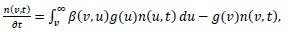(18)

by selecting the initial solution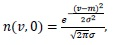the ADM uses the following recursion relation to generate components of the solution as: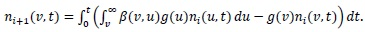(19)

Consequently, we can write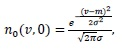(20)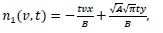(21)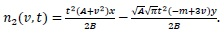(22)

Then, we find the solution by the general term as: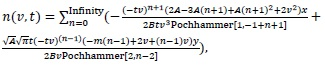(23)

with the following closed solution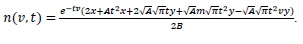(24)

Case 2: Breakup in a batch stirred vessel with a uniform daughter droplets distribution and parabolic breakup frequency with respect to the droplet volume.

By using the same algorithm which was used in Case 1 we get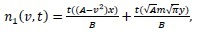(25)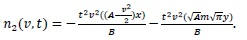(26)

The solution for this case is: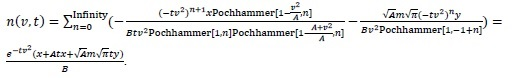(27)

Case 3: Breakup in a continuous flow with a uniform daughter droplets distribution and linear breakup frequency with respect to the droplet volume.

Starting with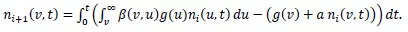(28)

Then we have with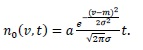(29)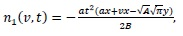(30)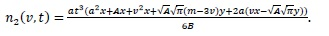(31)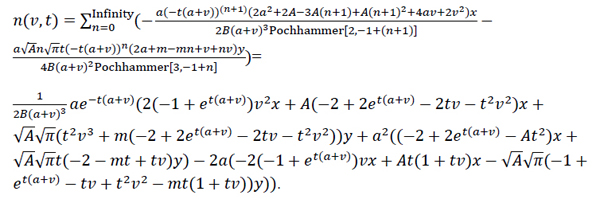(32)

Case 4: Breakup in a continuous flow with a uniform daughter droplets distribution and parabolic breakup frequency with respect to the droplet volume.

With the same algorithm in Case1 we obtain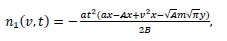(33)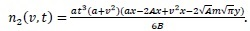(34)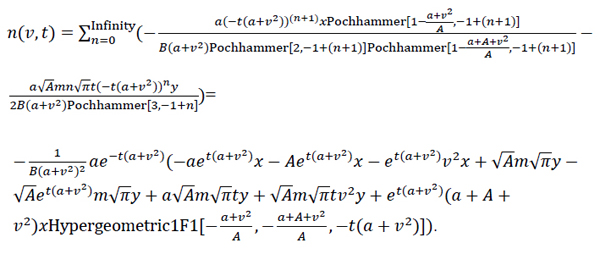(35)

With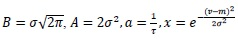and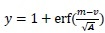, where m, σ and τ are the mean, standard deviation and the residence time for all cases, respectively.

Case 5: Breakup in a continuous flow with a physically relevant problem.

The inlet feed distribution, initial condition, daughter droplets distribution and breakup frequency are given in Table 1.

In Fig. (1), the complete droplets size distribution and the corresponding zeroth and third order moments, that are the total number and the total mass of droplets, respectively, are shown for the uniform daughter droplets distribution and linear breakup frequency in batch process with an initial condition supposed normal (Gaussian) distribution with mean m=3 and standard deviation σ=0.3. It is clearly shown from this figure that the zeroth moment increases, since more and more droplets are produced and the third moment is constant as expected by mass conservation. The prediction of the droplets size distribution as well as its related moments is very good accurate by both the finite element method with expansion coefficients based on the Chebyshev polynomials and the obtained analytical solution by the decomposition method.

Fig. (2) presents the complete droplet size distribution and the corresponding zeroth and third-order moments for the uniform daughter droplets distribution and parabolic breakup frequency in a batch process with an a Gaussian distribution initial condition with of the same mean and a standard deviation as in Case 1. The prediction of the droplet size distribution as well as its related moments is very good accurate by both the finite element method with expansion coefficients based on the Chebyshev polynomials and the analytical solution.

## 3. RESULTS

The numerical results presented in this article show that the present numerical method conserves the zeroth and third moments well enough.

In Fig. (3), both analytical and numerical determined droplet size distribution and its corresponding moments for the initial distribution condition equal zero and the feed distribution are assumed a normal Gauss distribution with m = 3 and σ = 0.3 in a continuous flow with a uniform daughter droplets distribution and linear breakup frequency have been plotted. This figure includes the both the solutions for the variation of the zeroth and third-order moments. Once more, the agreement is excellent by the finite element method with expansion coefficients based on Chebyshev polynomials and the analytical solution.

Fig. (4) Once again, with m=3 and σ=0.3 for the Gauss feed distribution, the results for the droplet size distribution by the finite element method with the expansion coefficients based on the Chebyshev polynomials and the analytical solution for the Case 4 are accurate.

Fig. (5) presents numerical results corresponding to the physically relevant example as indicated in Table 1, with mean droplet volume μ = 0.5 10-9m3 and σ = 0.625 10-10m3 for the water-toluene system, and the agitation energy input εm = 0.15W/kg. In case 5, the analytical results are not available. We have therefore plotted only numerical results computed by the finite element method. The excellent agreement found between the present results and the previous predictions of  clearly illustrates the physical and numerical consistency of the present numerical method.

Fig. (6) shows the comparison of the relative merits of different discretization orders. We first present the solutions obtained with the number of degrees of freedom Ndof=96. There are 96 bins for the polynomial of order zero, 48 bins for the polynomial of order 1, 32 bins for the polynomial of order 3, and 24 bins for the polynomial of order 3.

Therefore, the consistency of the numerical results can be improved by expanding the order of the polynomial.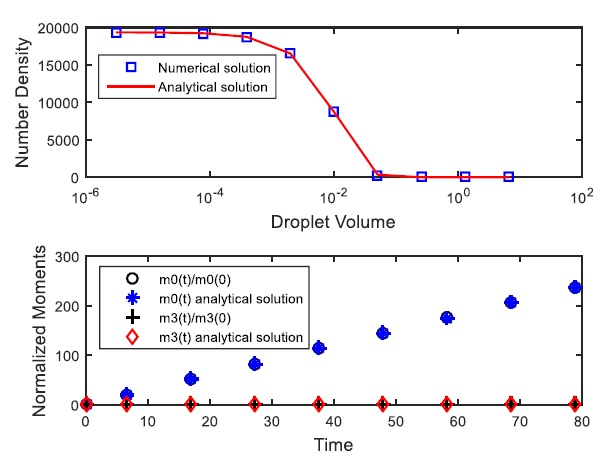Fig. (1). A comparison of numerical and analytical results in Case 1.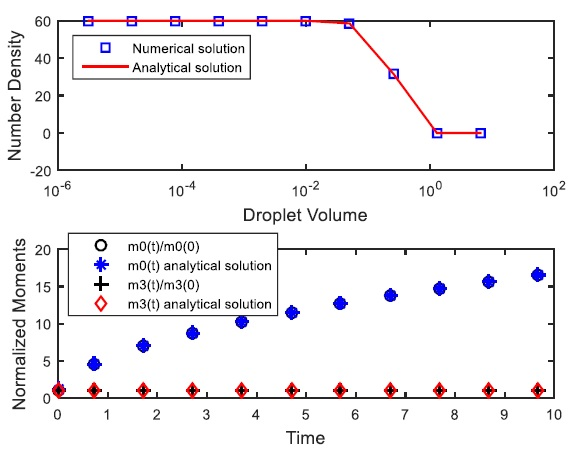Fig. (2). A comparison of numerical and analytical results in Case 2.Fig. (3). A comparison of numerical and analytical results in Case 3.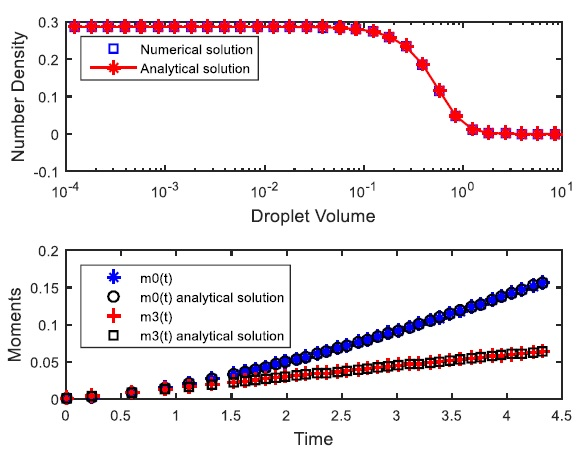Fig. (4). A comparison of numerical and analytical results in Case 4.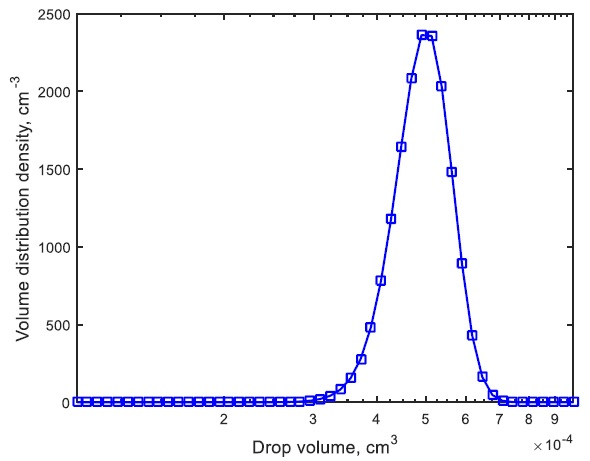Fig. (5). Time evolution of drop volume distribution density at 1200 s for Case 5.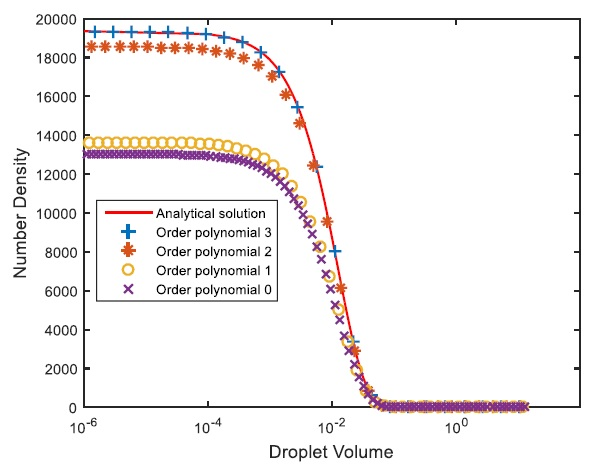Fig. (6). The solutions for the Case 1 obtained with Ndof = 96 degrees of freedom.

The accuracy of the numerical solutions at the final time using these four polynomials of order zero, 1, 2, and 3 is obtained against the analytical solution by the root-mean-square error norm whose errors are 0.2478, 0.2240, 0.0318 and 0.0011, respectively.

## CONCLUSION

The droplet breakup in batch and continuous flow is described by the population balance equation (PBE), which represents the particle size distributions in a particulate process. The PBE is solved analytically and numerically. The proposed numerical method of the finite element method with expansion coefficients approach based on Chebyshev polynomials is employed to solve such equations with effectiveness and accuracy when compared to the exact solutions obtained by the Adomian decomposition method. The advantage of this present numerical method is that it does not require the reconstruction of the distribution from moments, unlike the original quadrature method of moments and its numerous extensions. With the proposed method, the construction of the distribution function and the moments is obtained straightforward.

The extension of the present numerical method to more complex systems, such as bubbly flows, requires further extension.

Not applicable.

Not applicable.

### FUNDING

This work was supported by DFG (Deutsche Forschungsgemeinschaft, SPP 1740, Project 256646572) working.

### CONFLICT OF INTEREST

The authors declare no conflict of interest, financial or otherwise.

Declared none.

## REFERENCES

  Ramkrishna D. Population Balances 2000.  Randolph A, Larson M. Theory of particulate processes 1971.  Cameron I, Wang F, Immanuel C, Stepanek F. Process systems modelling and applications in granulation: A review. Chem Eng Sci 2005; 60: 3723-50.  Dhanasekharan K, Sanyal J, Jain A, Haidari A. A generalized approach to model oxygen transfer in bioreactors using population balances and computational fluid dynamics. Chem Eng Sci 2005; 60: 213-8.  Lage PLC. On the representation of qmom as a weighted-residual method-the dual-quadrature method of generalized moments. Comput Chem Eng 2011; 35: 2186-203.  Marchisio DL, Fox RO. Solution of population balance equations using the direct quadrature method of moments. Aerosol Sci 2005; 36: 43-73.  Hasseine A, Meniai AH, Bencheikh Lehocine M, Bart H-J. Assessment of drop coalescence and break-up for stirred extraction columns. Chem Eng Technol 2005; 28: 552.  Hasseine A, Bellagoun A, Bart H-J. Analytical solution of the droplet breakup equation by the adomian decomposition method. Appl Math Comput 2011; 218: 2249-58.  Hasseine A, Senouci S, Attarakih M, Bart H-J. Application of two analytical approaches for the solution of the population balance equations: Particle breakage process. Chem Eng Technol 2015; 38: 1574-84.  Hasseine A, Bart H-J. Adomian decomposition method solution of population balance equations for aggregation, nucleation, growth and breakup processes. Appl Math Modelling 2015; 39: 1975-84.  Alzyod S, Attarakih M, Hasseine A, Bart H-J. Steady state modeling of kühni liquid extraction column using the spatially mixed sectional quadrature method of moments (SM-SQMOM). Chem Eng Res Des 2017; 117: 549-56.  Liou JJ, Srienc F, Fredrickson AG. Solutions of population balance models based on a successive generations approach. Chem Eng Sci 1997; 52: 1529-40.  Mahoney AW, Ramkrishna D. Efficient solution of population balance equations with discontinuities by finite elements. Chem Eng Sci 2002; 57: 1107-19.  Nicmanis M, Hounslow MJ. A finite element analysis of the steady state population balance equation for particulate systems: Aggregation and growth. Comput Chem Eng 1996; 20: 261-6.  Rigopoulos S, Jones AG. Finite-Element scheme for solution of the dynamic population balance equation. AIChE J 2003; 49: 1127-39.  Sandu A, Borden C. A framework for the numerical treatment of aerosol dynamics. Appl Numer Math 2003; 45: 475-97.  Bennett MK, Rohani S. Solution of population balance equations with a new combined lax–wendroff/crank–nicholson method. Chem Eng Sci 2001; 56: 6623-33.  Bove S, Solberg T, Hjertager BH. A novel algorithm for solving population balance equations: The parallel parent and daughter classes. derivation, analysis and testing. Chem Eng Sci 2005; 60: 1449-64.  Chang SC. The method of space–time conservation element and solution element -a new approach for solving the navier–stokes and Euler equations. J Comput Phys 1995; 119: 295-324.  Filbet F, Laurençot P. Numerical Simulation of the Smoluchowski Coagulation Equation, SIAM J. on Sci. Comp 2004; 25: 2004-28.  Motz S, Mitrovic A, Gilles ED. Comparison of Numerical Methods for the Simulation of Dispersed Phase Systems. Chem Eng Sci 2002; 4329-44.  Verkoeijen D, Pouw GA, Meesters GMH, Scarlett B. Population Balances for Particulate Processes—a Volume Approach. Chem Eng Sci 2002; 57: 2287-303.  Kumar S, Ramkrishna D. On the Solution of Population Balance Equations by Discretization – I. A Fixed Pivot Technique. Chem Eng Sci 1996a; 51: 1311-32.  Kruis FE, Maisels A, Fissan H. Direct Simulation Monte Carlo Method for Particle Coagulation and Aggregation. AIChE J 2000; 46: 1735-42.  Lee K, Matsoukas T. Simultaneous Coagulation and Breakage using Constant-N Monte Carlo. Powder Technol 2000; 110: 82-9.  Lin Y, Lee K, Matsoukas T. Solution of the Population Balance Equation using Constant-Number Monte Carlo. Chem Eng Sci 2002; 57: 2241-52.  McGraw R. Description of Aerosol Dynamics by the Quadrature Method of Moments. Aerosol Sci Technol 1997; 27: 255-65.  Attarakih M, Drumm C, Bart H-J. Solution of the population balance equation using the sectional quadrature method of moments (SQMOM). Chem Eng Sci 2009; 64: 742-52.  Yuan C, Laurent F, Fox R. An Extended Quadrature Method of Moments for Population Balance Equations. J Aerosol Sci 2012; 51: 1-23.  Attarakih M, Bart H-J, Abu-Khader M. On the solution of the population balance equation: From global to local constrained maximum entropy method. Chem Eng Sci 2019; 209115168  Adomian G. Solving Frontier Problems of Physics: The Decomposition Method 1994.  Adomian G, Rach R. On linear and nonlinear integro-differential equations. J Math Anal Appl 1986; 113: 199-201.  Ribeiro LM, Regueiras PFR, Guimaraes MML, Madureira CMN, Cruz-Pinto JJC. The dynamic behaviour of liquid-liquid agitated dispersions. I-The Hydro- dynamics. Comput Chem Eng 1995; 19: 333-43.